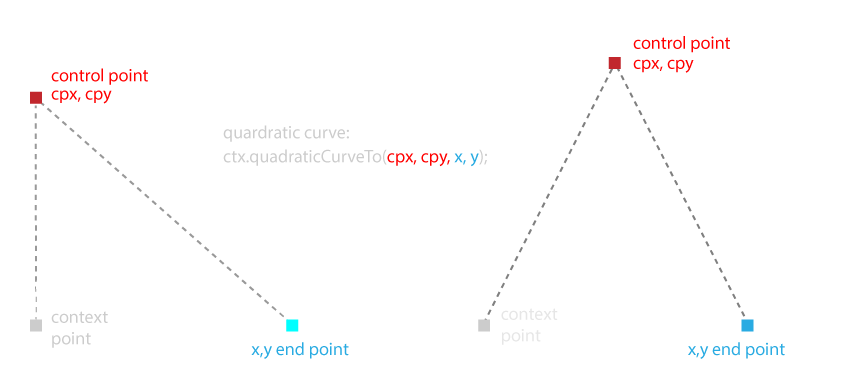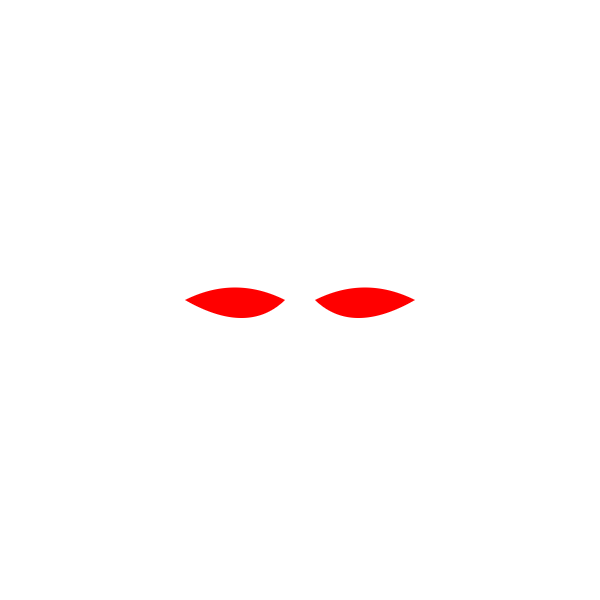## Canvas - Drawing Bamboo with Code

After drawing rectangles, lines, circles (arcs) and triangles, about all that’s left in the toy box is the curve. Canvas has two types of curves: quadratic and cubic; they differ only in the amount of control points used for bending the line.

Quadratic curves have two anchor points and one control point and curves in only one direction. The more complex cubic curve adds another control point and can curve in two directions.

Curves can be interwoven when drawing paths with lines and arcs, and they pick up wherever the current context point is (or from a explicit moveTo which established a new context point):

``ctx.quadraticCurveTo(cpx, cpy, x, y);``

where cpx and cpy is the control point and x,y is the endpointPlaying with this:

``// w = canvas.width// h = canvas.heightctx.clearRect(0, 0, w, h);ctx.save();ctx.translate(w / 2 + 15, h / 2);ctx.beginPath();ctx.moveTo(0, 0);ctx.quadraticCurveTo(50, -25, 100, 0);ctx.quadraticCurveTo(37, 36, 0, 0);ctx.fill();//ctx.setTransform(1,0,0,1,0,0); //reset matrixctx.translate(w / 2 - 15, h / 2);ctx.scale(-1, 1); // mirror image...ctx.beginPath();ctx.moveTo(0, 0);ctx.quadraticCurveTo(50, -25, 100, 0);ctx.quadraticCurveTo(37, 36, 0, 0);ctx.fill();ctx.restore();``

The code draws two curves connected to each other (then endpoint on the second was the starting point on the first curve); the second set of curves calls scale(-1,1) before repeating the same calls again and creates a mirror image, which results:Using curves for eyes and leaves in the next project here’s an experiment constructing a bamboo grove. Each time the canvas is clicked it regenerates a new grove.

See the Pen Triangle Spirals by Kentskyo (@kentskyo) on CodePen.

Previous Canvas - Triangles Next Canvas - Animation